# CH 610A/618A

First Exam

Fall, 2003

Prof.N.L.Bauld

__________________________________________                                                                              Name (Last, First)

__________________________________________                                                                            Social Security Number

## Possible Points

Page/Pts/Points Earned

## Score

I.

22

2/10/

3/12/

II.

23

4/10/

5/9/

6/4/

III.

22

7/10/

8/12

IV.

13

9/8/

10/5/

V.

20

11/11/

12/9/

Totals

100

### I.Ground State Electronic Configurations and Hybridizations

A.  Electronic Configurations.

1.   [2 pts] Write the ground state electronic configuration of positively charged nitrogen (7N +) in the energy level diagram format.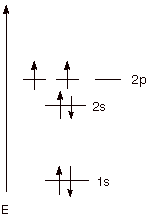1.    [4 pts] Name the three rules which are needed to derive the correct ground state configuration and explain how they are applied to the case of positively charged nitrogen atoms.

q      The Aufbau Rule: Fill in electrons beginning with the lowest energy orbital first and proceeding to orbitals of progressively higher energy.In the above case, electrons go into the 1s orbital first, then the 2s, then the 2p orbital.

q      The Pauli Exclusion Principle: A maximum of two electrons can occupy any orbital but their spins must be paired. Two electrons can go into the 1s and 2s orbitals, if their spins are paired.

q      The Hund Rule: In the case of degenerate (equienergetic) orbitals, the lowest energy state is provided by filling electrons into different orbitals rather than into the same orbital, and with their spins unpaired, i.e., having the same spin. The fifth and sixth electrons then go into different 2p AO’s, but they have the same spin.

B.  Electronic Configuration and Hybridization of Carbon.

1.   [4 pts] Using the linear format only, write the ground state electronic configuration of positively charged carbon atoms (6C +) and show in stepwise fashion (two steps) how positively charged carbon atoms hybridize in carbocations (e.g., methyl carbocation, CH3+). Be sure to include (1) the names of any other states involved in addition to the ground state (2) the potential valence of carbon in that state and (3) comment on the energetic feasibility of each of the individual steps and of the overall process of hybridization to relevant final state.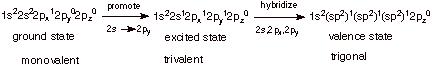q      The promotion of a 2s electron to a 2py orbital requires about 96 kcal/mole of energy (ca. 100 kcal/mol is close enough). The increase in valency from 1 to 3, provides two extra bonds at ca. 100 kcal/mol apiece. Consequently, the increased number of bonds more than supplies the need for energy to promote the electron.

2.     [1 pt] Which neutral atom has the same ground state electronic configuration as positively charged atomic carbon? Why?

q      Boron, because it also has 5 electrons.

3.    [2 pts] What is the name of the principle which interrelates positively charged atomic carbon and this neutral atom? What does this principle predict about the hybridization state of this neutral atom when bonded to three hydrogen atoms?

q      The isoelectronic principle.

q      It predicts that boron will have a trigonal hybridization, the same as carbon, in this situation.

4.    [4 pts] Draw an energy level diagram which shows the energies of the 2s and 2p atomic orbitals of atomic carbon. Then place the energies of the sp, sp2, and sp3 hybrid orbitals on the same diagram, using care to display the energies of these latter orbitals not only in the right energy sequence but with approximately the right spacings between these hybrid orbitals and in relationship to the 2s and 2p AO energies.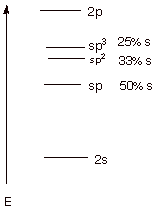5.    [2 pts]What percentage of s character is in each of these three hybrid AO’s? Indicate how this percentage is derived.

q      See diagram above.

q      An sp3 AO means s1p 3. This AO has 3 parts 2p and 1 part 2s character, so it is 25% s and 75% p.

C. Alkenes

1.    [2 pts] Starting with the ground state electronic configuration of neutral carbon, sketch the stepwise development of the hybridization state of carbon in ethyne (acetylene; C2H2 ) and other alkynes. What is the designation of this hybridization state?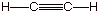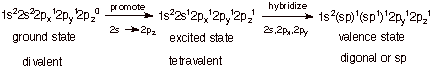2.    [1 pt] When (under what general circumstances) does neutral carbon adopt this hybridization state?

q      When it is only bonded to two other atoms.

### II. Atomic Orbitals, Molecular Orbitals, and Bonding

#### A. Carbon-Carbon Bond Formation

1. The overlap of two carbon 2p AO’s in the manner shown below can result in the formation of a strong covalent bond.

a.   [3 pts] Referring to the diagram given below, label the two new orbitals which are formed when these two atomic orbitals overlap, providing both the abbreviations and the full names of the orbitals. Explain the specific basis for the names assigned to them.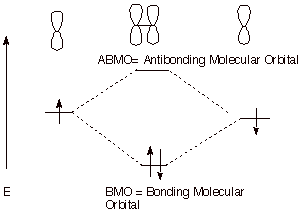q      The reference point is the energy of the starting atomic orbital. An MO of energy more negative than this is bonding; an MO having an energy less negative than this (higher in energy) is antibonding.

b.   [2 pts] Explain why the two electrons both go into the same orbital. According to what principle must their spins be paired? What unallowable circumstance would necessarily result if (somehow) the third electron were added (not just a violation of the rule above).

q      The Pauli Exclusion Principle requires the spins to be paired

q      If two electrons having the same spin (spins unpaired) went into the same orbital, we would have two indistinguishable electrons. (For a similar reason, we cannot have three electrons in the same orbital, because necessarily two of the elelctrons would have to have the same spin.

c.   [2 pts] Given the geometric nature of the overlap depicted in the diagram above, what kind of covalent bond is this? What kind of overlap is involved in this kind of bond?

q      Pi overlap (or lateral overlap)

q      A Pi Bond.

d.   [3 pts] Provide a depiction of both of the new orbitals which are formed in the bond formation process indicated above in terms of their constituent 2p orbitals. Include the signs of the lobes and any nodal planes. In terms of a specific type of overlap, what is different about the low energy orbital and the high energy orbital?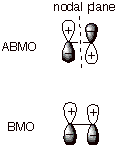q      The BMO has only positive overlap; the ABMO has only negative overlap.

e. [2 pts] Compare the relative efficiency of the type of overlap shown above and found in ethene, with the type which is found in ethane. What is the name of the type of overlap found in ethane?

q      The overlap in ethane is of the sigma type (end-on overlap). This is much more efficient than the pi (lateral) overlap in the pi bond of ethene.

2. A bond line structure for ethene is given below. The following questions refer to the bonding in ethene.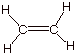a. [2 pts] Provide an orbital depiction of all of the sigma bonds in ethene (carbon-hydrogen and carbon-carbon).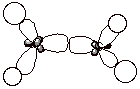b. [2 pts] Explain why all six atoms lie in the same plane and why rotation around this bond involves breaking a bond. Use Newman projection structures to depict your answer.

q      Both carbons are sp2 hybridized and individually coplanar with the three attached groups (H,H, and C).

q      The trigonal planes of the two trigonal carbons are additionally required to be coplanar because only in that way is efficient pi overlap effected.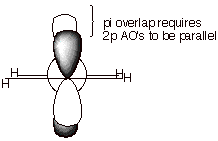B. Strong and Weak Covalent Bonding.

1.   [3 pts] Specify the general requirements for strong covalent bonding, briefly explaining the basis for each requirement.

q      Efficient (or strong), net overlap.

q      Two paired electrons.

q      Since only one bonding MO is formed it can accommodate a maximum of 2 electrons, and then only if there spins are paired (Pauli Exclusion). Bonding is maximized if this low energy orbital is fully occupied (2 e).

q      In general, the stronger the overlap, the lower the energy of the BMO, so strong overlap gives more bonding. Net overlap refers to the possibility that there can be strong overlap in one region, but negative overlap in another, and the two may cancel, yielding no net overlap.

2.   [2 pts] Cite two specific situations in which atoms closely approach and efficient orbital overlap results, but no covalent bonding at all results. The situations should be fundamentally different. Provide energy level diagrams illustrating each of these cases.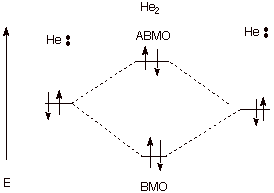q      In the case of the close approach of two helium atoms, strong overlap occurs and BMO/ABMO formation occurs, but there are four electrons (each He has two electrons), so that the amount of antibonding which ensues from the ABMO cancels the bonding which would have been accrued through the BMO.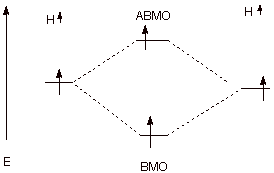q      In the case of close approach of two hydrogen atoms which both have the same spin (spins unpaired), there are two electrons, but they cannot be put into the BMO. One must, by the Pauli Principle, occupy the ABMO, again negating the bonding effect of the BMO.

3.   [2 pts] Cite two specific situations in which atoms closely approach and

efficient orbital overlap occurs, but only weak or modest covalent bonding results. Again, the two situations should be fundamentally different. Provide energy level diagrams illustrating each of these cases.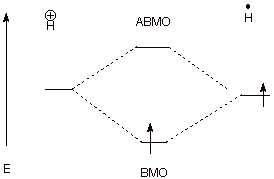q      The close approach of a proton to a hydrogen atom leads to strong overlap and BMO/ABMO formation, but there is only one electron to place in the BMO, so that net, but moderate, bonding occurs.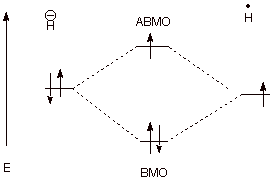q      In this case, a hydride anion (which has a hydrogen nucleus with two electrons) approaches a hydrogen atom. There is weak, net bonding because there are two electrons in the BMO and only one in the ABMO, so there is not full cancellation of the bonding effect.

### III. Nomenclature, Formal Charges, and Resonance Structures

A.  Formal Charges.

1.   [5 pts] Calculate the formal charges on each atom other than hydrogen in the following structures. Provide the formula you are using, and show your calculations. Then, calculate the net charge on the species.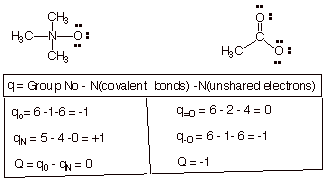q      Where Q is the net charge, and q is the charge on an atom; q=O is the charge on the doubly bond oxygen, etc.

B.  Resonance Structures.

1.   [2 pt] Indicate whether the structures shown below are valid resonance structures, and explain why or why not.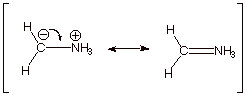q      The first structure is valid—all valency rules are satisfied for carbon and nitrogen, but the second structure is not. The nitrogen atom is pentacovalent, thus violating the octet rule.

2.   [2 pts] Using the appropriate formalism of resonance theory, describe the acetate ion (one canonical structure is shown below) in terms of resonance theory.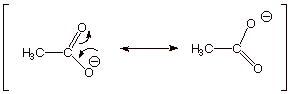3.   [1 pt] Summarize this as a dotted line/partial charge structure.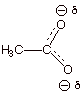4.   [1 pt] The resonance energy of the acetate anion is characterized as large. Upon what basic principle of resonance theory is this based?

o      Resonance stabilization is particularly large when the resonance structures are equivalent in energy.

5.   [1 pt] What is the main geometric consequence of resonance in the acetate anion?

q      The two C-O bonds are equal in length and intermediate between single and double.

6.   [1 pt] Provide an orbital depiction of the pi electron system of the acetate anion. Such an extended (more than two AO’s are involved) pi electron system is termed a conjugated system. Over how many atomic orbitals are the MO’s of this conjugated system delocalized?

q      Delocalization of the electrons is over three atoms, the two oxygens and the carbonyl carbon.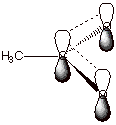C. Nomenclature

1. [3 pts each = 6 pts] Provide complete, accurate IUPAC names for the following molecules. As a part of your answer, include the numbering system and the basis for your choice of numbering system.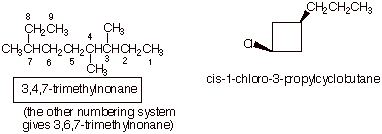q      In the second structure, the carbon attached to chlorine is numbered as 1 because of alphabetics (chlorine is treated just like an alkyl substituent).

q      In that same structure, the molecule is treated as a cyclobutane derivative , rather than a cyclobutylpropane derivative, because the propyl group has only three carbons, whereas the cylcobutane ring has four.

3.    [3 pts] Provide the IUPAC name for the following molecule, using the appropriate IUPAC substituent name.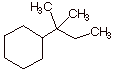q      1,1-dimethylpropylcyclohexane.

IV. Conformational Analysis; Torsional Strain; Steric Effects.

A.  Conformational Analysis of Butane.

1.   [5 pts] Sketch an energy vs. dihedral angle conformational energy plot for butane, providing the names, Newman projection structures, and relative energies of all conformational extrema.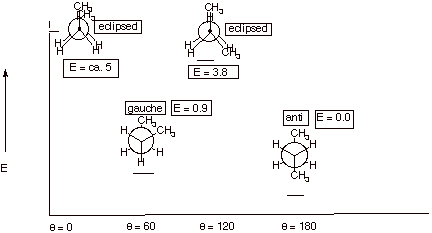2.   [1 pt] What is the name of the type of effect which accounts for the difference in energies of the two energy minima of butane. Describe the fundamental basis for this effect and provide a depiction of it.

q      Steric effect or van der Waals repulsion.

q      A repulsion between hydrogen atoms of the methyl groups which are too close, i.e., closer than the sum of the van der Waals radii.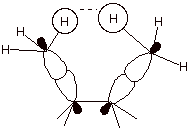3.   [2 pts] Explain the difference between torsional strain and steric strain , using a depiction to assist in illustrating your answer.q      Steric strain derives from a repulsion between atoms which are too close, while torsional strain derives from the repulsions of electron pairs in bonds, which are closer in the eclipsed than in the staggered conformation.

C.  Ring Strain.

1.   [3 pts] Using the diagram provided below as a starting point for your illustration, describe and illustrate the basis for the angle strain present in cyclopropane. Be certain to include the concepts of interorbital angle and internuclear angle in your explanation.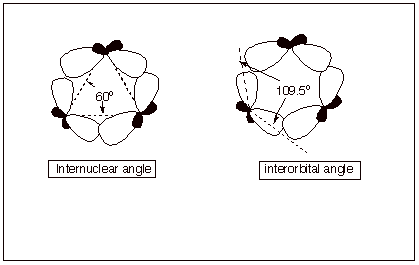q      The internuclear angle for an equilaterial triangle is 60­­ degrees, but the interorbital angle for sp3 orbitals on the same atom is the tetrahedral angle.

q      The result of this non-correspondence is that the orbitals on the ring carbons can not point toward each other, as is the case for a typical, strong carbon-carbon sigma bond. The bond is “bent”.

2.   [2 pts] What other type of strain is present in cyclopropane? Explain the basis for this strain, and indicate which bonds are responsible for it.

q      Cyclopropane also has torsional strain because, being planar, all of the C-H bonds are eclipsed.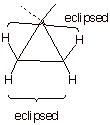V. Conformations of Cyclohexane and Substituted Cyclohexanes.

A. Cyclohexane Conformational analysis.

1. [2 pts] Draw the conformational structure of the conformation of cyclohexane which represents the energy minimum. Show clearly on your structure and label the axial and equatorial carbon-hydrogen bonds (all of them).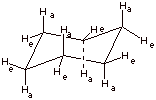2. [3 pts] How much strain is present in this conformation of cyclohexane? Explain your answer in detail, with reference to both types of strain which are typically present in cycloalkanes.

q      Zero strain.

q      There is no torsional strain because all of the C-H and C-C bonds are staggered.

q      There is no angle strain because all of the C-C-C angles are 109.5 degrees.

3. [1 pt] Draw the structure of this energy minimum conformation again and then show (connected by a equilibrium arrows) the structure which would result upon “ring flip”. (Do not show the detailed conformational path at this point, but just the final ring flip structure.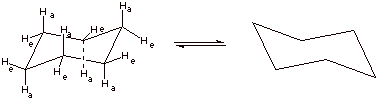4. [2 pts] Draw and name the structure of the global energy maximum on the ring flip path, and indicate its energy relative to the minimum energy structure. What is the importance of this structure?q      This is the “half chair” conformation.

q      Its energy is 10 kcal/mol greater than that of the chair

q      The importance is that this is the highest point on the ring flip path and determines the activation energy and therefore the rate of the flip.

B. Monosubstituted Cyclohexanes.

1.    [3 pts] Draw the conformational structure of the conformation of methylcyclohexane which is of lowest energy and show the structure which results upon ring flip. Provide the names and relative energies of these two structures.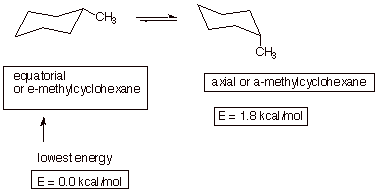2. [2 pts] Using a depiction to assist in clarifying your answer, explain which structure is the global minimum, what type of strain is present in the less stable conformation, and the basis for the strain.

q      As noted, the equatorial conformation is more stable.

q      The axial conformation has steric strain or diaxial strain. Alternatively, it can be described as the equivalent of two gauche butane interactions.

q      This arises from the hydrogens of the methyl group repulsing each of the other two axial hydrogens on the same same of the generalized plane.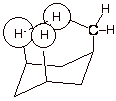C. Disubstituted Cyclohexanes.

1. [1 pt each = 6  pts] Provide complete IUPAC names for and specify quantitatively how much strain is present in each of the following disubstituted cyclohexanes and indicate what type of strain it is. Which of the three structures is the most thermodynamically stable?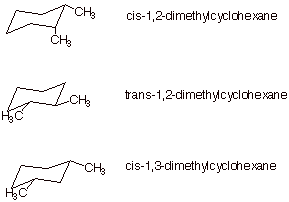q      The first structure has one axial methyl group, so it has 1.8 kcal/mol of 1,3-diaxial strain.

q      The second structure has no axial methyls, but the two methyl groups are gauche to one another. It has 0.9 kcal/mol of gauche butane strain.

q      The third structure has no axial methyl groups and NO strain at  all.

q      The third structure is the most stable.

2.    [1 pt] Draw the ring flip conformer of the third (last) structure and comment on its thermodynamic stability?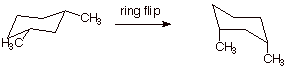q      This second conformer is highly unstable. It has an axial methyl interacting (not with an axial hydrogen, but) with an axial methyl group. The steric repulsions are very large!